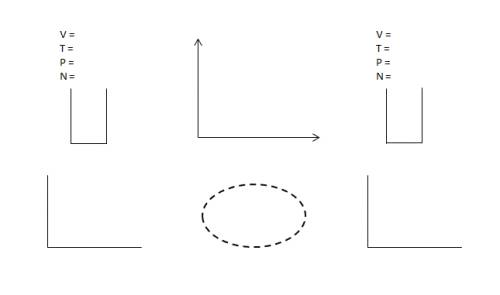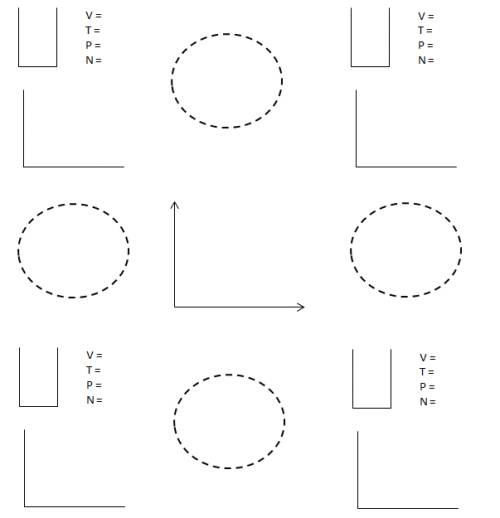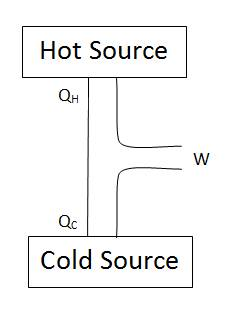# AP2 Unit 2: System of Ideal Particles Model

### Model Development

To begin this unit, we perform three experiments that together make up the paradigm lab. The first is connecting a syringe to a pressure sensor. Students record the volume from the scale on the syringe and note the pressure at that volume. As they then compress the plunger, they record the new volume and pressure.

The second experiment is to see how temperature effects pressure. The teacher notes show using a metal container. I couldn’t find one, so I used a glass bottle. Put a two hole stopper into the opening. In one opening, insert a digital thermometer, in the other, a valve to connect the pressure sensor. (Note: if you can’t get the thermometer to make a tight seal, you can put it in the water bath.) Now take the bottle and (partially) submerge into boiling water. I used a clip attached to a ring stand to hold the bottle just above the bottom of the beaker. I had the beaker of boiling water on top of a cold hotplate with a magnetic stirrer at the bottom of the beaker. Record the temperature and pressure, and then add ice. Wait until the temperature and pressure reach equilibrium, and repeat.

I was originally going to start with ice water and heat it up on the ice plate, but was told over the summer by a teacher at my AP workshop that the stopper will likely pop off due to the increase in pressure. That is why you start from boiling and cool down.

The third experiment is a little trickier. Attach a two hole stopper to a rigid container (again, I used the glass bottle). In one opening, the valve to the pressure sensor, in the other to a three-way valve that can be connected to the syringe and open to the atmosphere. Fill the syringe to a certain volume (I used 20 mL). Open the valve between the syringe and the container (closed to the air) and inject the air into the container. Close the valve to the container, such that is open to the atmosphere, and fill the syringe again to 20 mL. Repeat several times.

The end result of these three experiments should show graphs similar to what was seen in the computer modeling experiment:$Pressure \propto \frac{1}{Volume}$

The graph of pressure versus temperature is linear, with a positive intercept. This can lead to a discussion as to why the computer model was proportional, but the experiment was linear. The teacher should lead the students to the ideal of absolute zero. If you extrapolate the line, you can find the horizontal intercept which should be near$~ -273 ^oC$. If you translate the temperature into units of kelvin, you should then see that:$Pressure \propto Temperature$

and since the amount of gas added each time was constant, you can lead students to the conclusion that you were adding a constant number of particles each trial. The data should show a linear relationship. Once again, the teacher can lead the students to the conclusion that the vertical intercept represents the number of particles in the container at the beginning. If you were able to start with an empty container, then:$Pressure \propto Number$

From there, the teacher can lead the students to the combined proportionality seen in the computer simulation:$P \propto \frac {N T}{V}$

Although the computer simulation took about two weeks, I think it is worth it, as it gives the students a concrete visual model of what is happening. Most of my students have already taken both Chemistry and AP Chemistry. Although they already knew the equation for the Ideal Gas Law, most commented that it finally makes sense after working through this entire progression of the computer simulation and experiments.

### Model Deployment

##### Worksheet 1

The first worksheet has the students practice using the two equations developed in the simulation and experiments:$P = \frac{kNT}{V} = \frac {nRT}{V}$

and$U = \frac {3}{2} Nk_{B}T = \frac {3}{2} nRT$

It also introduces isothermal, isobaric, and isochoric processes. To analyze these, the worksheet introduces students to what I called Super LOL Diagrams which look something like this:The bottom row is the LOL you know and love from the Energy Transfer Model. (If you don’t know and love it, let me introduce you to Kelly O’Shea and her awesome explanation of LOL diagrams).

The only flavor of energy present in our system is the thermal energy we developed in the computer model:$U = E_{therm} = \frac {3}{2} k_{B}NT = \frac {3}{2} nRT$

The top row of the Super LOL is new. I hope to write post showing how to use this in greater detail (edited: see explanation of Super LOL here), but the short version is this:

Students should write all the values of the state variables (n or N, P, V, and T) where provided. They can use the Ideal Gas Equation if not all are given in the problem. The “U” shaped object below the state variables is a blank piston. Students shade in a qualitative picture of the piston before and after whatever process is occurring.

The “L” in between is for a$P-V$ graph. With this, students can determine the amount of working done by finding the area underneath the path from state 1 to state 2. The pistons help reinforce the direction of working, if compressed the surrounding is working on the system. If the piston is expanding, the system is working the surrounding.

In the “O” of the LOL diagram, the system is the gas inside the piston. In addition to working, the teacher introduces heating as a second energy exchange that can occur between the system and the surrounding.

With this, we have a visual tool to represent the First Law of Thermodynamics:$\Delta U = Q + W$

I’m holding off adding radiating as a third energy exchange until we get to light later in the course. I also am not going into conduction vs convection as I’m not sure how much this will come up. The AP equation sheet has the equation for conduction, but I guess we’ll see over the next few years how much it is used.

##### Worksheet 2

The second worksheet two builds upon the thermal processes in worksheet 1 by analyzing thermodynamic cycles. The Super LOL expands to become a Super LOL Box (I hesitate to call it the Super-Duper LOL, but maybe that’s a better name).The center “L” is the$P-V$ graph, the four corners are for each of the 4 stages in the cycle (You can make a LOL triangle for a 3 stage cycle or just cross out one corner of the box). The “O” in between is for the transition between those two adjacent steps of the cycle.

From here, the 2nd worksheet has students add up the total working in all the steps ($W$), the total heating added in all the steps ($Q_H$), and the total heating removed in all the steps ($Q_C$ into a diagram of the overall energy flow:##### Worksheet 3

Before attempting worksheet three, students are provided a reading that introduces 5 classic definitions of Entropy with proofs that they are all equivalent. It also introduces several of the common thermodynamic cycles commonly called Carnot Cycles and Otto Cycles. It also explains the equation for the change in Entropy:$\Delta S = \frac{Q_H}{T_c} - \frac{Q_H}{T_h}$

One of the things I like about the teacher notes is that they stress explaining Entropy as the spreading out of energy into the different degrees of freedom of the system.

With my students we discussed the $\frac {3}{2}$ in the thermal energy equation that more complex particles (aka molecules) will have a greater fraction (e.g.: \$latex \frac {5}{2} for diatomic molecules). I did that at the time of the lab this year, but may hold off until this point of the model deployment in the future.

By this logic, Entropy is similar to diffusion studied in chemistry (at least at our school it is) and heating in that the quantity flows from high concentration to low concentration. I think this makes Entropy easier to understand than the classic concept of Entropy as the “disorder” of the system.

In the worksheet, the students are led to see the similarities to heating in that we can try to predict what will happen as Entropy can only flow from high concentration to low, never the other way. If you need it to flow the opposite way, you must have a second process that provides work, and in the process has (an equal or) a greater flow of Entropy, what is commonly called the Second Law of Thermodynamics.